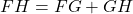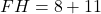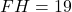## Point G is on line segment FH. Given FG = 8 and GH = 11, determine the length FH​

Question

Point G is on line segment FH. Given FG = 8 and GH = 11, determine the length FH​

in progress 0
3 weeks 2021-08-26T05:06:12+00:00 1 Answers 0 views 0

1. Given:

Point G is on line segment FH. FG = 8 and GH = 11.

To find:

The length of FH​.

Solution:

Point G is on line segment FH. So, by segment addition property, we getSubstitute FG = 8 and GH = 11.Therefore, the length of FH​ is 19 units.